Dr. J's Maths.com
Where the techniques of Maths
are explained in simple terms.

Applications of Calculus - basic rates of change.
Test Yourself 2.

 The questions on this page focus on: given a quantity; given a rate of change.

 Given the quantity. 1. A pool is being drained and its capacity in L litres of water at time t minutes is given by L = 120 (40 - t)2. (i) At what rate is the water draining out of the pool at t = 6 minutes? (ii) How long will it take for the pool to be completely empty? Answer.(i) Initial mass = 200 kg.(ii)Time = 35 mins. (iii) Rate = -40/7 (or -5.71) kg/min at 11.25 secs. 2. 3. 4.   Answer.(i) 23.1%.(ii)Peaks at 2 weeks. 5. Answer.Rate = (10π)/3 cm3/sec. 6. 7. 8. 9. 10. Given the rate. 11. A chemical factory is obliged to install special filtering equipment in accordance with the Clean Air legislation. At present the rate of emission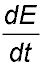in tonnes/month of pollution from the factory is given by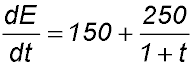where t is the time in months from the date at which the new filtering system was installed. (i) What is the rate of emissions when the equipment had just been installed? (ii) What will be the ultimate rate of emissions? (iii) Calculate the total amount of emissions (to the nearest tonne) to pollute the atmosphere by the end of the first year? Answer.(i) 400 tonnes.(ii) 100 tonnes.(iii) 2,078 tonnes. 12. A flat circular plate is being heated so that the rate of increase of its area - A m2 - after t hours is given by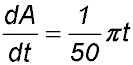Initially the plate has a radius of 5 metres. Express all answers in exact form. (i) What is the initial area of the plate? (ii) Develop an expression for the area of the plate as a function of t. (iii) What is the radius of the plate after 5 hours? (iv) How long does it take for the plate to increase in surface area by 20%? Answer.(i) Area = 25π.(ii) Area = 0.01πt2 + 25π.(iii) Radius = √(101)/2(iv) Time = 10√5; hours. MSC 2003 Q 8c MSC 2005 Q 8b MSC 2008 Q8b MSC 2007 Q10a MSC 2006 Q10a and MSC 2016 Q14d CSSA 1995 Q4c 13. A student attaches an air pressure pump to his bike tyre to inflate the tyres. The rate of change of the pressure in the tyre is given bypressure units per second. where t is the time after pumping has started. After 4 seconds of pumping, the pressure is 15 units. (i) What is the initial pressure in the tyre? (ii) The bike tyre will burst when the pressure reaches 40 units of pressure. At what time does this occur (answer to the nearest second). 20. The rate of fall in the price \$P of shares in a particular company is given by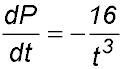where t is the number of months after the shares were listed on the stock exchange. One month after listing, the shares were selling for \$24. (i) Show that the price of the company's shares can be expressed by the relationship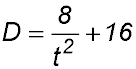(ii) Find the value of the shares after 2 months from listing. (iii) Find the rate at which the share price is falling after 3 months. (iv) Show that there is a minimum price for the shares and find that price. Answer.((ii) P = \$18.(iii) Rate = -\$0.59 per month.(iv) Minimum price is \$16.